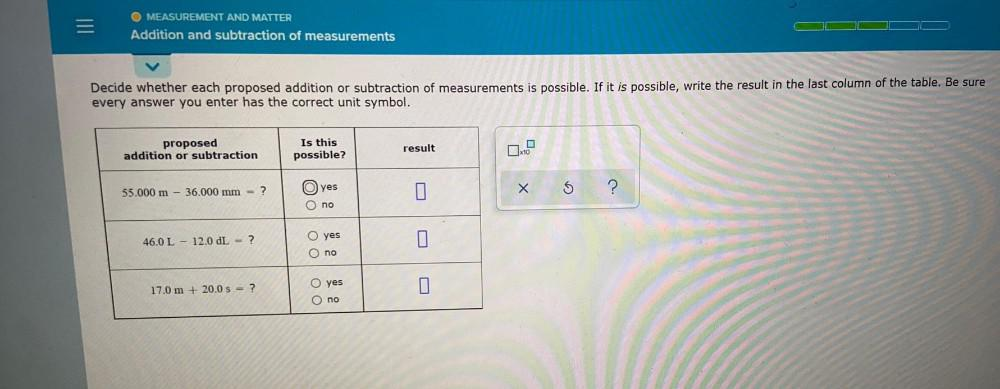Question:

# = MEASUREMENT AND MATTER Addition and subtraction of measurements Decide whether each proposed addition or subtraction of measur= MEASUREMENT AND MATTER Addition and subtraction of measurements Decide whether each proposed addition or subtraction of measurements is possible. If it is possible, write the result in the last column of the table. Be sure every answer you enter has the correct unit symbol. proposed addition or subtraction Is this possible? result G 55.000 m - 36.000 mm = ? O) yes X S ? no yes 46.0 L - 12.0 L = ? Oo O no U 17.0 m + 20.0 s = ? O yes O no